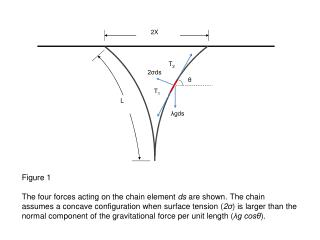Download Presentation2X

2X

Télécharger la présentation2X

- - - - - - - - - - - - - - - - - - - - - - - - - - - E N D - - - - - - - - - - - - - - - - - - - - - - - - - - -
Presentation Transcript

1. T2 2σds θ T1 λgds 2X L Figure 1 The four forces acting on the chain element dsare shown. The chain assumes a concave configuration when surface tension (2σ) is larger than the normal component of the gravitational force per unit length (λgcosθ).

2. T2 T1 2X L0 2σ ds λ g ds θ Figure 2 When the normal component of the gravitational force per unit length (λgcosθ) exactly balances the force per unit length due to surface tension (2σ), the chain assumes a perfect triangular shape.

3. X T2 2σds θ T1 λgds L Figure 3 When the normal component of the gravitational force per unit length (λgcosθ) is greater than the surface tension (2σ), the chain is pulled outward and assumes a concave configuration.

4. X T2 2σds θ T1 λgds L The equilibrium condition for chain element ds: dT= λgsinθds, (1) ( dT = T2 - T1 ) T dθ + 2σ ds = λgcosθds (2)

5. The governing differential equations for the chain: dT= λgsinθds, (1) ( dT = T2 - T1 ) T dθ + 2σ ds = λgcosθds (2) Deviding Eq. (1) by Eq. (2), dT/T = dθ (sinθ)/(cosθ - α) (3) (α ≡ 2σ/λg) Integrating Eq. (3), T = λg C/(cosθ –α) (4) (C is a constant) And finally the governing differential equation is: ds/dθ = C/(cosθ –α)2 (5)

6. T2 T1 2X L0 2σ ds λ g ds θ Equations For the Triangular Configuration: dT= λgsinθds, (1) ( dT = T2 - T1 ) 2σ ds = λgcosθds (2) (2σ = λgcosθ) Integrating Eq. (1) gives tension along the chain, T = λgsinθs (3)